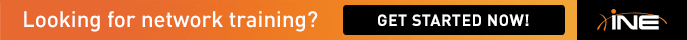# Task 8.3 two color policing

Hi guys, I don't understand how the bc, pir and be where calculated in this task, I checked at cisco and the examples there double cir to calculate pir, and I cannot find the formula to calculate the bc or be, the task mentioned a 30ms interval so I used the same formula as in traffic shaping but the results are different from the task.

police cir 512000 bc 1920 pir 896000 be 3360

http://www.cisco.com/en/US/docs/ios/12_3/qos/command/reference/qos_o1g.html#wp1084420

• Bc = Tc * CIR

So Bc = 0.03 * 512000, which equals 15360. Policing wants a value in bytes, so Bc (bytes) = 15360/8 = 1920.

PIR=CIR + EIR, which you can use to calculate the other values.

Or at least I think that's how it goes. What results where you getting?

Try reading the task breakdown of VOL1 "10.44 MQC Two-Rate Three-Color Policer"

• Thank you guys, I missed that bc and be are byte values.

Thanks,

• police cir 512000 bc 1920 pir 896000 be 3360

I am wondering if the following would do the same or not

police cir 512000 bc 1920 be3360

Any ideas ?

I thought burst excess would be only excess tokens. ie EIR*Tc so that PIR = (Bc+Be) * Tc
I probably missed something... & I definitevely need to go back to my studies on this point [:(]

•

Hi,

police cir 512000 bc 1920 pir 896000 be 3360 is Two-rate three-color policing. police cir 512000 bc 1920 be3360 is single-rate three-color policing.

HTH,

Bassam